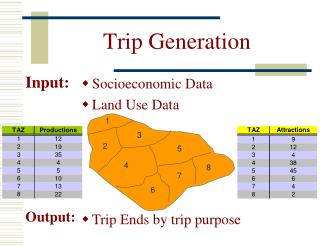DownloadDownload PresentationTrip Generation

# Trip Generation

Télécharger la présentation## Trip Generation

- - - - - - - - - - - - - - - - - - - - - - - - - - - E N D - - - - - - - - - - - - - - - - - - - - - - - - - - -
##### Presentation Transcript

1. 1 3 2 5 4 8 7 6 Trip Generation Input: • Socioeconomic Data • Land Use Data Output: • Trip Ends by trip purpose

2. Trip Generation Trip Distribution The question is… how do we allocate all the trips among all the potential destinations? Trip MatrixorTrip Table Zone 1

3. Trip Distribution

4. Trip Distribution • We link production or origin zones to attraction or destination zones • A trip matrix is produced • The cells within the trip matrix are the “trip interchanges” between zones

5. Basic Assumptions of Trip Distribution • Number of trips decrease with COST between zones • Number of trips increase with zone “attractiveness”

6. Methods of Trip Distribution • Growth Factor Models • Gravity Model

7. Growth Factor Models • Growth Factor Models assume that we already have a basic trip matrix • Usually obtained from a previous study or recent survey data

8. Growth Factor Models • The goal is then to estimate the matrix at some point in the future • For example, what would the trip matrix look like in 2 years time? Trip Matrix, T (2018) Trip Matrix, t(2008)

9. Some of the More Popular Growth Factor Models • Uniform Growth Factor • Singly-Constrained Growth Factor • Average Factor • Detroit Factor • Fratar Method

10. Uniform Growth Factor Model

11. Uniform Growth Factor i = I = Production Zone j = J = Attraction Zone Tij = τ tij for each pair i and j Tij =Future Trip Matrix tij = Base-year Trip Matrix τ = General Growth Rate

12. Tij = τ tij for each pair i and j Tij =Future Trip Matrix tij = Base-year Trip Matrix τ = General Growth Rate Uniform Growth Factor If we assume τ = 1.2 (growth rate), then… Trip Matrix, t(2008) Tij = τ tij = (1.2)(5) = 6 Trip Matrix, T (2018)

13. Uniform Growth Factor • The Uniform Growth Factor is typically used for over a 1 or 2 year horizon However, assuming that trips growat a standard uniform rate is a fundamentally flawed concept

14. The Gravity Model

15. The Inspiration for the Gravity Model The big idea behind the gravity model is Newton’s law of gravitation… The force of attraction between 2 bodies is directly proportional to the product of masses between the two bodies and inversely proportional to the square of the distance M1 M2 F = k r2

16. Some of the Variables Tij = Qij = Trips Volume between i & j Fij =1/Wcij = Friction Factor Wij = Generalized Cost (including travel time, cost) c = Calibration Constant pij = Probability that trip i will be attracted to zone j kij = Socioeconomic Adjustment Factor Tij = Trips between i & j i = production zone j = attraction zone Tij = f (Pi, Aj, Cij) Cij = Generalized cost of trip from i to j

17. The Gravity Model The bigger the friction factor, the more trips that are encouraged Pi Aj FijKij Tij =Qij = = Pipij ΣAjFijKij (Productions)(Attractions)(Friction Factor) = Sum of the (Attractions x Friction Factors) of the Zones Fij = 1 / Wcij & ln F = - c ln W

18. To Apply the Gravity Model What we need… • Productions, {Pi} • Attractions, {Aj} • Skim Tables {Wij) • Target-Year Interzonal Impedances

19. Gravity Model Example 8.2 • Given: • Target-year Productions, {Pi} • Relative Attractiveness of Zones, {Aj} • Skim Table, {Wij} • Calibration Factor, c = 2.0 • Socioeconomic Adjustment Factor, K = 1.0 • Find: • Trip Interchanges, {Qij}

20. Find Trip Interchanges, {Qij} Find Denominator of Gravity Model Equation {AjFijKij} Calculate Friction Factors, {Fij} Find Probability that Trip i will be attracted to Zone j, {pij} Target-Year Inter-zonal Impedances, {Wij} Given… 1 F11= = 0.04 52 AjFijKij Calibration Factorc = 2.0 Socioeconomic Adj. FactorK = 1.0 Given… Calculate Friction Factors, {Fij} 1 1 Fij = = Fij = Wcij Wcij Find Denominator of Gravity Model Equation {AjFijKij} AjFijKij=A4F34K34 (5)(0.01)(1.0) = 0.05 Find Probability that Trip i will be attracted to Zone j, {pij} 0.05 pij = = 0.3125 = Σ(AjFijKij) 0.16 Find Trip Interchanges, {Qij} Qij = Pipij = (2600)(0.3125) = 813

21. Keep in mind that the socioeconomic factor, K, can be a matrix of values rather than just one value

22. The Problem with K-Factors • Although K-Factors may improve the model in the base year, they assume that these special conditions will carry over to future years and scenarios • This limits model sensitivity and undermines the model’s ability to predict future travel behavior • The need for K-factors often is a symptom of other model problems. • Additionally, the use of K-factors makes it more difficult to figure out the real problems

23. Limitations of the Gravity Model • Too much of a reliance on K-Factors in calibration • External trips and intrazonal trips cause difficulties • The skim table impedance factors are often too simplistic to be realistic • Typically based solely upon vehicle travel times • At most, this might include tolls and parking costs • Almost always fails to take into account how things such as good transit and walkable neighborhoods affect trip distribution • No obvious connection to behavioral decision-making